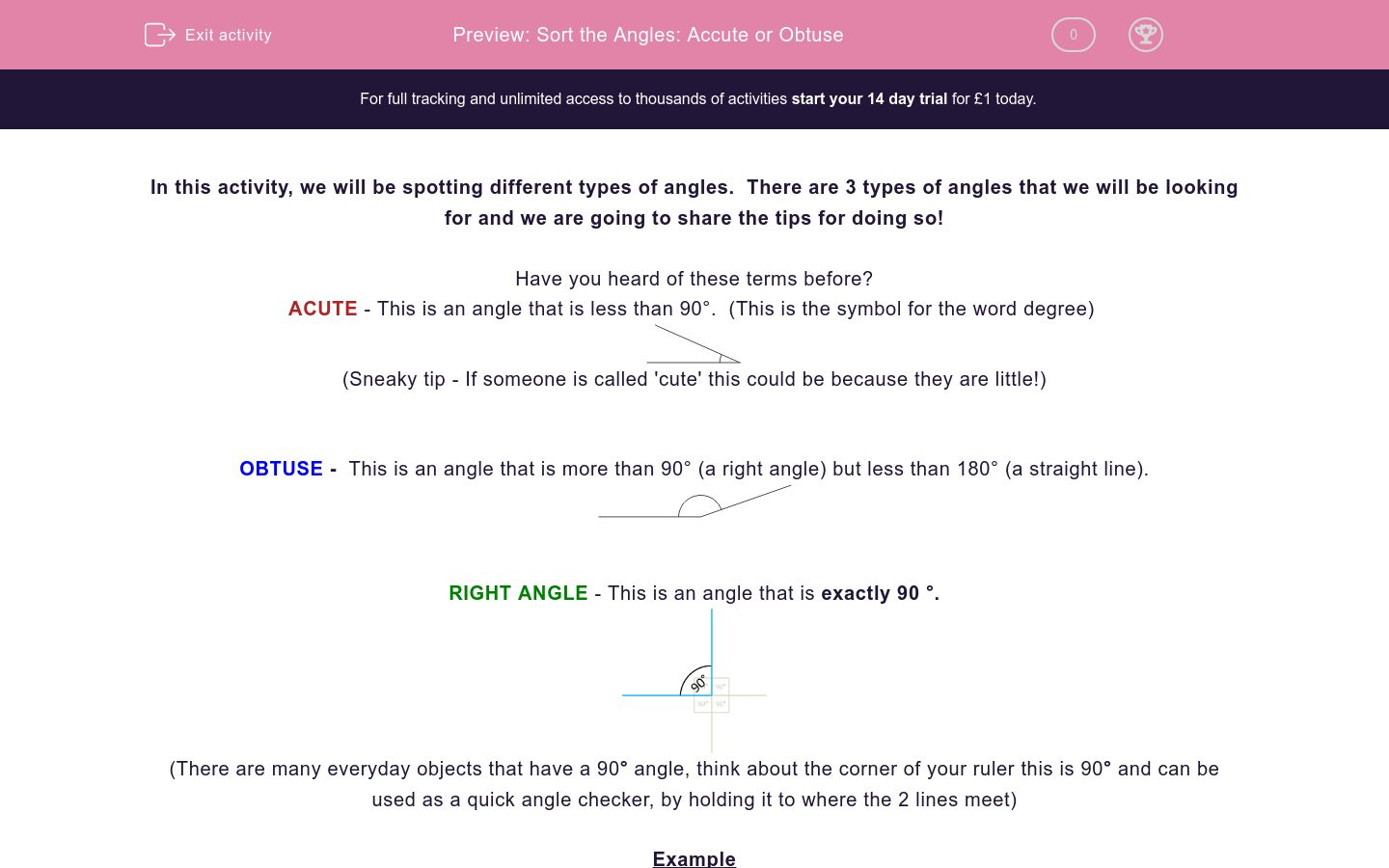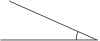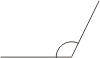# Sort the Angles: Accute or Obtuse

In this worksheet, students sort angles into acute or obtuse.Key stage:  KS 2

Curriculum topic:   Maths and Numerical Reasoning

Curriculum subtopic:   2D Shapes: Circles, Angles and Bearings

Difficulty level:### QUESTION 1 of 10

In this activity, we will be spotting different types of angles.  There are 3 types of angles that we will be looking for and we are going to share the tips for doing so!

Have you heard of these terms before?

ACUTE - This is an angle that is less than 90°.  (This is the symbol for the word degree)(Sneaky tip - If someone is called 'cute' this could be because they are little!)

OBTUSE -  This is an angle that is more than 90° (a right angle) but less than 180° (a straight line).RIGHT ANGLE - This is an angle that is exactly 90 °.(There are many everyday objects that have a 90° angle, think about the corner of your ruler this is 90° and can be used as a quick angle checker, by holding it to where the 2 lines meet)

Example

Sort the angles into acute and obtuse.

(a)(b)(c)(d)(e)(f)Acute angles: c, d, e.

These are less than 90° (a right angle)

Obtuse angles: a, b, f.

These are more than 90° (a right angle) but less than 180° (a straight line)

Sort the angles into acute and obtuse.

(a)(b)(c)(d)(e)(f)a b c d e f Acute Obtuse

Sort the angles into acute and obtuse.

(a)(b)(c)(d)(e)(f)a b c d e f Acute Obtuse

Sort the angles into acute and obtuse.

(a)(b)(c)(d)(e)(f)a b c d e f Acute Obtuse

Sam has labelled this angle as an acute angle in his maths test.  Do you think Sam is correct or not?

(a)Correct

Incorrect

Select the statements that are true below.

(a)(b)(c)(d)Half of the angles above are acute

Angle a) and d) are both obtuse

Angle a) and b) are the same type of angle

All angles above are the same type of angle

Have a look at the angle below.  Which of the statements is correct?

(a)Angle a) is greater than 90 degrees

Angle a) is smaller than 180 degrees

Angle a) is an acute angle

Label the angles below using one word (acute or obtuse).

(a)(b)Angle a) is greater than 90 degrees

Angle a) is smaller than 180 degrees

Angle a) is an acute angle

Sort the angles into acute and obtuse.

(a)(b)(c)a b c Acute Obtuse

Complete the sentence below.

 a b c Acute Obtuse

In a regular 2D square, what size are all of the angles?  If you think it may help, why not draw this out and look for a common theme among the angles.• Question 1

Sort the angles into acute and obtuse.

(a)(b)(c)(d)(e)(f)a b c d e f Acute Obtuse
EDDIE SAYS
Think about a corner of a page (this would be an example of a 90 degree angle) if an angle is bigger than this it will be obtuse. If it is smaller, it will be acute. Can you see that a, b and f are bigger than 90 degrees so therefore must be obtuse angles? In turn, c, d and e are smaller than 90 degrees. If you want to check it, hold a ruler at where the two lines meet. This will give you a 90 degree angle to check against.
• Question 2

Sort the angles into acute and obtuse.

(a)(b)(c)(d)(e)(f)a b c d e f Acute Obtuse
EDDIE SAYS
How did you do? Did you use your ruler to check? Hopefully, you can see that options a.b and c are smaller than 90 degrees, whereas, d,e and f are bigger so they must be obtuse angles.
• Question 3

Sort the angles into acute and obtuse.

(a)(b)(c)(d)(e)(f)a b c d e f Acute Obtuse
EDDIE SAYS
Are you getting the hang of this now? Remember to check if the angle is bigger than 90 degrees it will be obtuse, and anything smaller is acute. (Another tip is that an acute angle often looks a bit like the lead end of a pencil!)
• Question 4

Sam has labelled this angle as an acute angle in his maths test.  Do you think Sam is correct or not?

(a)Incorrect
EDDIE SAYS
Sam is incorrect as the angle in the image is greater than 90 degrees meaning that this angle is obtuse.
• Question 5

Select the statements that are true below.

(a)(b)(c)(d)Half of the angles above are acute
Angle a) and b) are the same type of angle
EDDIE SAYS
Firstly, you need to label each angle as either obtuse or acute. After that you should see that a) is obtuse b) is obtuse c) is acute d) is acute When you look at it like this you can then approach each of the statements and you will find that only 2 options are true. Half the angles above are acute (c and d). Angles a) and b) are the same type of angle, which is an obtuse angle.
• Question 6

Have a look at the angle below.  Which of the statements is correct?

(a)Angle a) is greater than 90 degrees
Angle a) is smaller than 180 degrees
EDDIE SAYS
What sort of angle is angle a)? It's an obtuse angle. This immediately removes the last sentence from being correct. Think back to what we know about obtuse angles. An obtuse angle is greater than 90 degrees (a right angle) but is smaller than 180 degrees (a straight line). Therefore the first 2 options must be correct.
• Question 7

Label the angles below using one word (acute or obtuse).

(a)(b)EDDIE SAYS
You'll be seeing angles everywhere you look! Angle a) is an obtuse angle as it is greater than 90 degrees. Angle b) you can see is smaller, and because it is less than 90 degrees is an acute angle.
• Question 8

Sort the angles into acute and obtuse.

(a)(b)(c)a b c Acute Obtuse
EDDIE SAYS
Always remember that 90 degrees is the magic number when working out angles! Does angle a) look bigger or smaller than 90 degrees? It looks smaller! So it has to be an acute angle. Angle b) is also smaller than 90 degrees so that's another acute angle. Angle c) that's clearly much bigger than the other 2, and definitely greater than 90 degrees. There you go, you've solved it!
• Question 9

Complete the sentence below.

EDDIE SAYS
An angle on a straight line is always 180 degrees. This is something to remember! If you think you may struggle, why not jot it down!
• Question 10

In a regular 2D square, what size are all of the angles?  If you think it may help, why not draw this out and look for a common theme among the angles.90 degrees
90
EDDIE SAYS
Did you get 90 degrees? That's right if you did! Remember that anything that looks like the corner of your ruler will be 90 degrees. All of the angles in a square are 90 degrees as they are all identical!
---- OR ----

Sign up for a £1 trial so you can track and measure your child's progress on this activity.

### What is EdPlace?

We're your National Curriculum aligned online education content provider helping each child succeed in English, maths and science from year 1 to GCSE. With an EdPlace account you’ll be able to track and measure progress, helping each child achieve their best. We build confidence and attainment by personalising each child’s learning at a level that suits them.

Get started